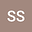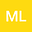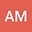Total Ionospheric Conductance: Summation of Sources
•••• +1## Abstract

Conductivity of the ionosphere allows the complex system of magnetospheric currents to flow through. Conductivity is governed by several factors including electron density and temperature, whose influence is highly dynamic during geomagnetic storm events. Thus, it is a crucial parameter that must be determined for space weather modeling to specify the coupling between the magnetosphere, ionosphere and thermosphere systems. Major sources of ionospheric conductivity are solar EUV and particle precipitation which includes Diffuse (Diff.), Monoenergetic (ME) and Broadband (BB) precipitations. Conductance Σ is the height integrated version of conductivity. Empirically, total ionospheric conductance (Hall and Pedersen) is known to be the root sum square of individual conductance terms [Wallis and Budzinski, 1981], considering that conductivity resulting from different processes are not linearly additive and corresponding ionization rates shall be added at each altitude and then integrated over the desired altitude range. With the inclusion of the less energetic broadband precipitation that was found to cause ionization in the bottom-side F region, the expression for the total ionospheric conductance was modified by the linear addition of the contribution of the broadband precipitation to the total Hall and Pedersen conductance[Zhang et al., 2015].In this study, using a 3-dimensional global physics based model GITM (Global Ionosphere Thermosphere Model), the validity of this combination of vector and linear addition of individual source terms to the total ionospheric conductance is examined and the more accurate expression for the summation of sources contributing to the total conductance is quantified. GITM is employed to calculate the Hall and Pedersen conductance using the average energy, potential and energy flux for each of the sources of conductance. Several scenarios are simulated where the different sources of precipitation are paired with solar EUV radiation, and the total conductance is obtained. Linear and vector summation of conductance resulting from combinations of sources and individual sources indicate that the contribution of broadband precipitation to the total conductance also follows vector addition. To quantify the result that the total conductance is the vector sum of individual sources, error histograms are plotted and a set of metrics including RMSE, mean error, standard deviation, correlation coefficient and fractional error are enumerated for both linear and vector summation of individual sources to produce the total conductance.